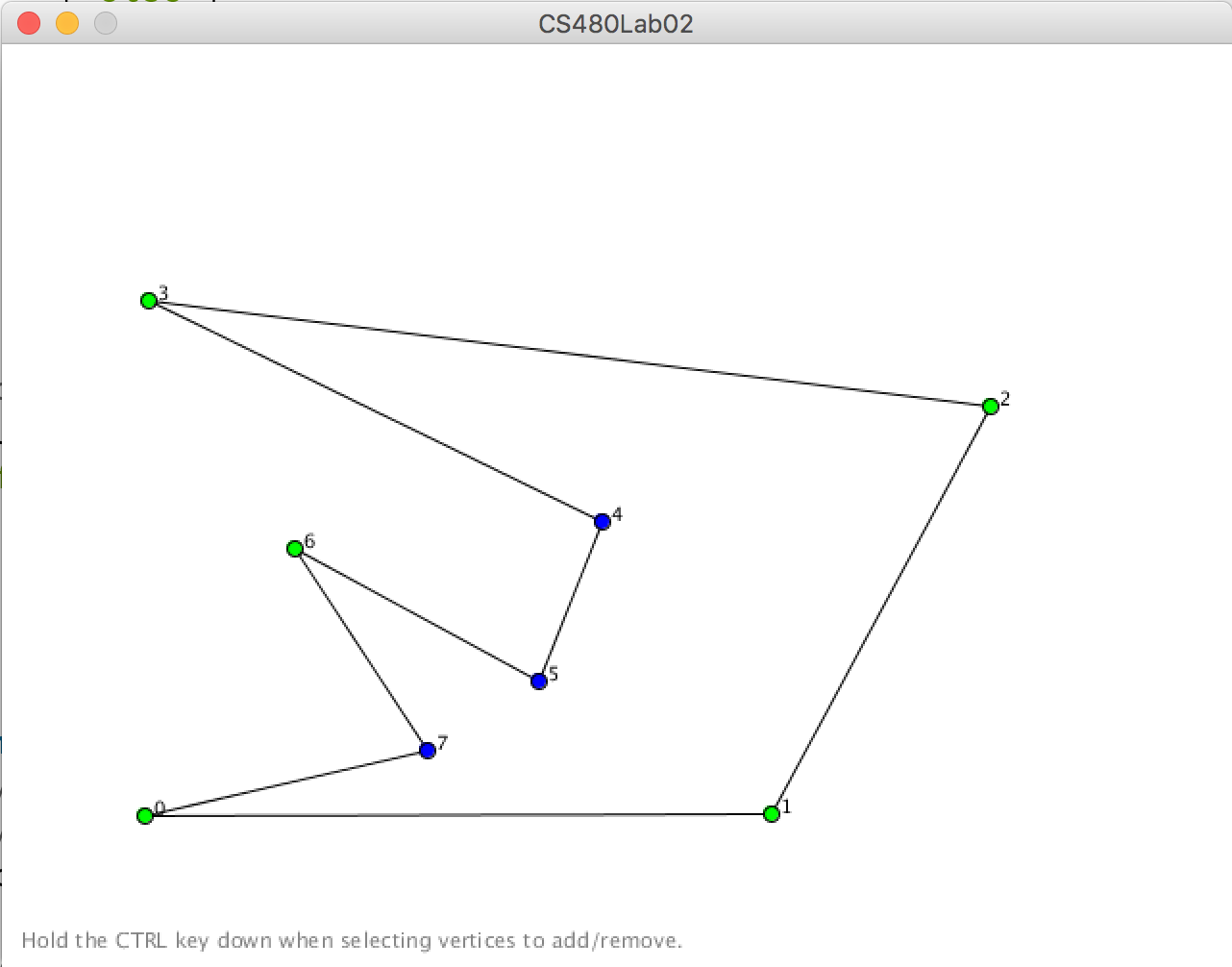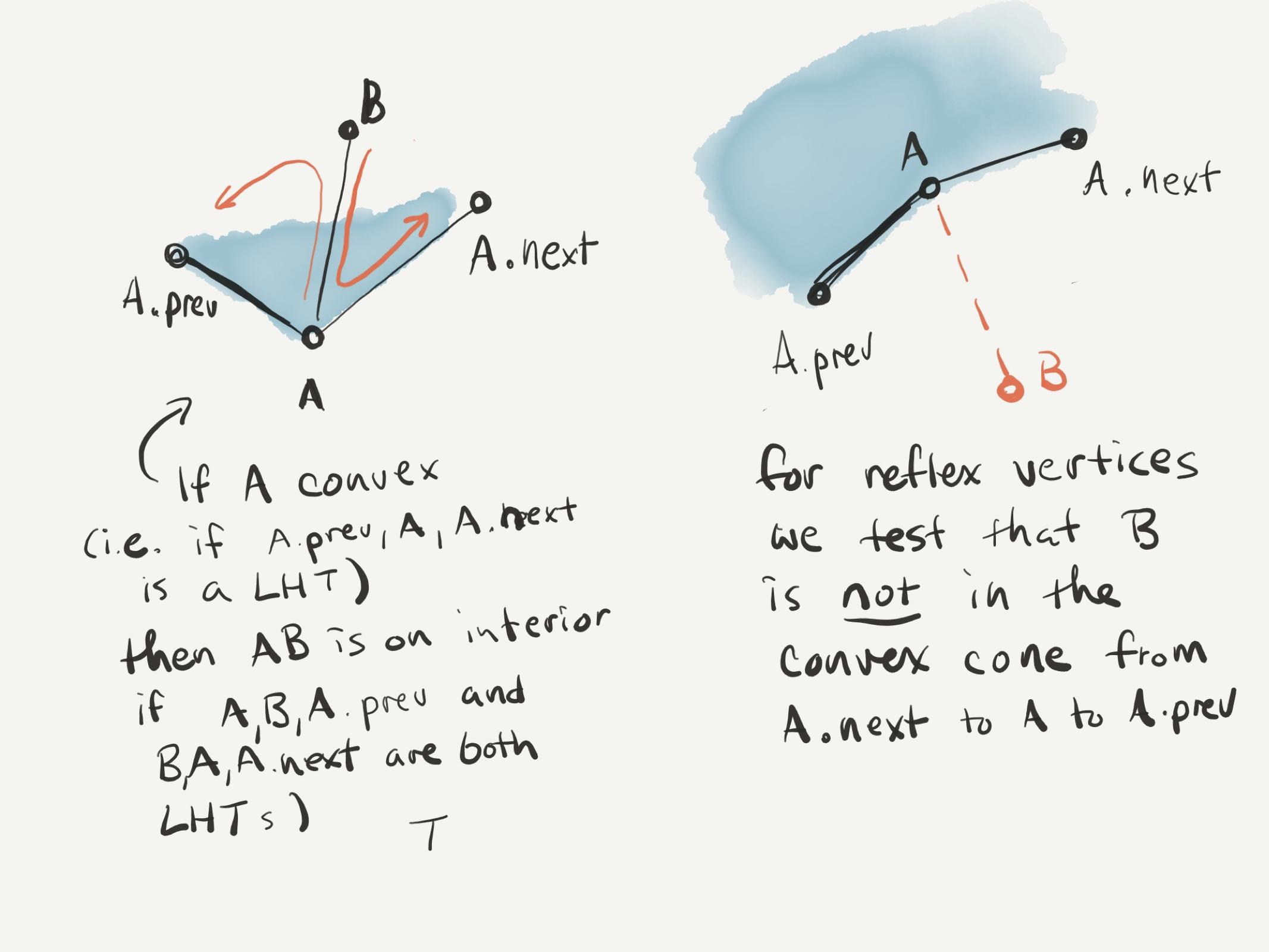# Introduction

The purpose of this lab is to implement a simple algorithm for triangulating the interior of a polygon. In the last lab you learned about the signed area of a triangle and explored several simple geometric predicates that can be constructed from it. Recall that using only the concept of signed area, we can determine whether three consecutive points form a left-hand or a right-hand turn, and then additionally, use left and right-hand turn tests to determine whether two line segments cross. Our triangulation code will build on these predicates to find a diagonal of a polygon. Once we have code for finding the diagonal of a polygon we will find a set of non-crossing diagonals that triangulates any input polygon.

Key Concepts

The following concepts will play a key role in today’s activity:

• Geometric predicates/primitives
• Triangulations
• Counter-clockwise vs. clockwise orientation

# Getting started

Download the starter code, unzip it, and open up the main Processing file CS480Lab02.pde in Processing.

The starter code is a simple polygon editor which looks like:The initial polygon is oriented counter-clockwise. A green vertex denotes a left-hand turn and a blue vertex denotes a right-hand turn. You can click and drag vertices to modify the polygon. You can also add/delete vertices by holding down the CTRL button when you click. Each vertex’s index in the polygon (counting from 0) is shown to the right of the vertex to aid you in debugging purposes.

# Storing a polygon

In the previous lab, we just stored a polygon as an array-based list of points. This is somewhat inconvenient for many algorithms, because we often want a vertex just before or just after some given vertex. Implementing this using array-based techniques requires that we also juggle indices into the array and correctly use the modulus operator to wrap around the end of the polygon. For this lab we store a polygon as a circular doubly-linked list of vertices. The main polygon class just keeps a reference to one “first” vertex, and the remaining vertices are accessed by following next and prev pointers to move from one vertex to the next or previous. The canonical loop for accessing all vertices looks like this:

Vertex v = polygon.firstVertex; // Start at the first vertex
do {
// Do something here with the vertex v.
v = v.next; // Move to the next vertex
} while (v != polygon.firstVertex); // Keep going until we end back where we started


Have a look through the drawing code to see how these loops are used to draw the polygon edges and vertices. Make sure you understand this code before continuing.

# Adding same side and segment crossing predicates

Your first task is to use the left-hand turn predicates (defined in the Predicates) file, to define two predicates. The first has the following signature:

boolean onSameSide(Point2i A, Point2i B, Point2i L0, Point2i L1)


This method should return true if A and B are on the same side of the line through points L0 and L1, false otherwise. Recall that this can be done using left-hand and right-hand turn tests.

Your next test is to determine whether two line segments (S0, S1) and (S2, S3) cross or not. Recall that we saw in class that the two segments cross if and only if S0 and S1 are on opposite sides of hte line through S2 and S3 and the points S2 and S3 are on opposite sides of the line through points S0 and S1. The signature for this method should be:

boolean crosses(Point2i S0, Point2i S1, Point2i S2, Point2i S3)


Return true if the two segments cross, false otherwise.

Finally, test your predicates by adding a method for checking whether the first edge and the third edge of the polygon are crossing to the Polygon class. The signature for this method is:

boolean isFirstAndThirdEdgeCrossing()


Add code to the draw() method to change the polygon’s edge color to red if the first and third edges are crossing. Run and test your code. Don’t continue until you’ve got this working.

Challenge Problem: Write code to determine whether any pair of edges are self-crossing instead of just the first and third edges.

# Detecting diagonals

You now have code for determining whether any two given line segments cross. Let’s now consider the problem of determining whether a pair of vertices $(A, B)$ of a polygon form a diagonal. Recall that a diagonal of a polygon is a pair of non-consecutive vertices $(A, B)$ with the following two properties: (1) the line segment from $a$ to $b$ does not cross or touch any of the other line segments in the polygon, and (2) the line segment $AB$ is on the interior of the polygon. You now have enough to write a test for the first property, but we also need to know whether the line segment $(A, B)$ is on the interior or exterior of the polygon.

Here we will assume that the polygon is oriented counter-clockwise. Notice from the figure below, that if the vertex $A$ is a convex vertex, we can determine whether $AB$ is inside the polygon by testing whether both $A, B, A.prev$ and $B, A, A.next$ are left-hand turns. Additionally, we can check whether $A$ is, in fact, convex by testing whether $A.prev, A, A.next$ forms a left hand turn. On the other hand, if $A$ is reflex, then this test will not work (can you show an example why?). Instead we use the following trick: flip the order of the polygon, i.e. consider $A.next$ to come before $A$ and $A$ to come before $A.prev$. We will now test whether $AB$ is not inside the polygon, by testing whether $A.next, A, B$ and $B, A, A.prev$ both form left-hand turns. The figure below illustrates this concept:Now, write a method as a member of the Polygon class

boolean isDiagonal(Vertex A, Vertex B)

which determines if the vertices $A$ and $B$ form a diagonal for the polygon. You may assume that the given $A$ and $B$ are in fact vertices of the polygon.

Test your method by adding some code to the draw() method to draw a blue diagonal between the first and third vertices whenever it exists.

# Computing a triangulation

Now that we know how to test whether a pair of vertices $(A, B)$ is a diagonal, we can use the following naive algorithm for computing a triangulation. Recall that a triangulation is given by any maximal set of non-crossing diagonals. This gives us the following algorithm: maintain a set of accepted diagonal. For each pair of non-consecutive vertices $(A, B)$, test whether $(A, B)$ is a diagonal. If it is, then test whether it crosses any of the already accepted diagonals. If it does not, accept it as another diagonal in the accepted set, otherwise, reject it. Once all diagonals have been tested, the accepted diagonals will constitute a triangulation.

You will now implement this method. The Polygon file includes a helper class Diagonal that stores a pair of points. Use it to add the following method to your Polygon class:

ArrayList<Diagonal> triangulate();


to return a list of diagonals that triangulates your polygon. Once you have this add drawing code to draw() to draw your triangulation (and color the triangulation’s diagonals something other than black.)

Then answer the following:

• What is the running time of this naive method?
• What happens if you flip the orientation of your polygon from counter-clockwise to clockwise? Why does this happen?

# Going further: a challenge

Here’s a faster method for triangulation. Select some three consecutive vertices $A$, $B$, and $C$. First, check whether $AC$ is a diagonal. If it is, split the polygon into two polygons by adding the diagonal and recurse on both sides. If it is not, find the vertex of the polygon that lies inside the triangle $ABC$ (why must such a vertex exist?) that is closest to $B$. Let’s call it $X$, you are guaranteed that $BX$ is a diagonal (again, why?). Split the polygon along $BX$ and recurse on both sides. Stop recursion whenever you hit a triangle.

• Code the algorithm above. You may want to add a split(A, B) method that returns a pair of polygons after splitting between $A$ and $B$.
• What is the running time of the algorithm?

# Turn it in

Turn it in via Canvas by Friday 11pm.